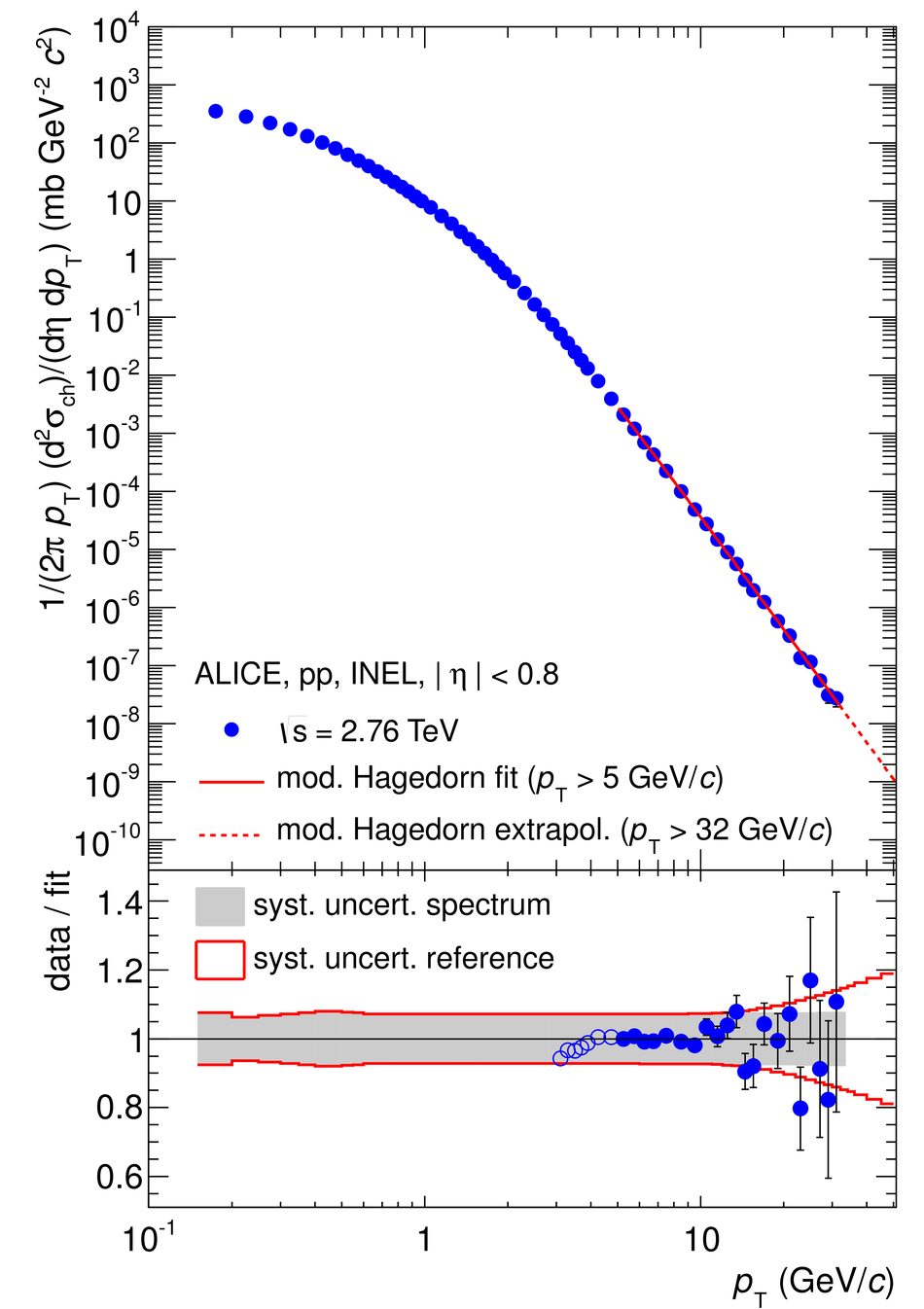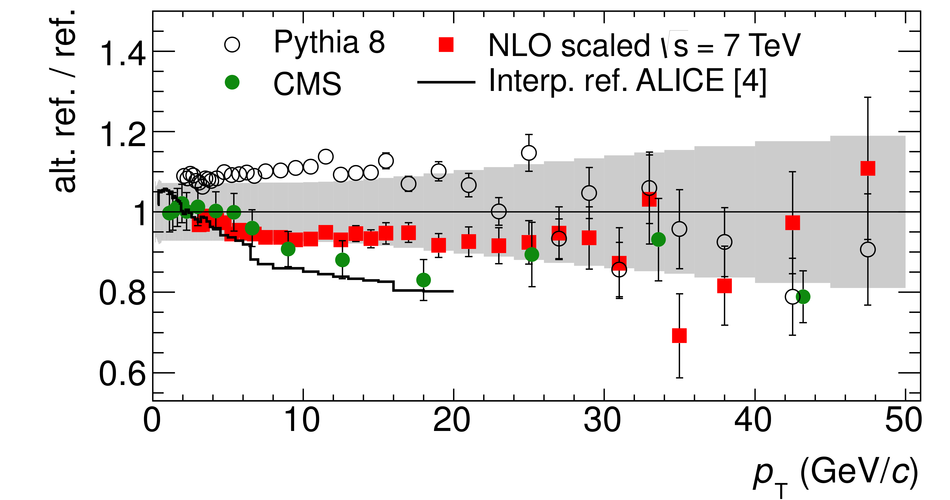# Energy Dependence of the Transverse Momentum Distributions of Charged Particles in pp Collisions Measured by ALICE

Differential cross sections of charged particles in inelastic pp collisions as a function of $\pT$ have been measured at $\sqrt{s} = 0.9$, 2.76 and 7 TeV at the LHC. The $\pt$  spectra are compared to NLO-pQCD calculations. Though the differential cross section for an individual $\sqrt{s}$ cannot be described by NLO-pQCD, the relative increase of cross section with $\sqrt{s}$ is in agreement with NLO-pQCD. Based on these measurements and observations, procedures are discussed to construct pp reference spectra at $\sqrt{s} = 2.76$ and 5.02 TeV up to $\pt = 50$ GeV/$c$ as required for the calculation of the nuclear modification factor in nucleus-nucleus and proton-nucleus collisions.

Figures

## Figure 1

 Top panel: Differential cross section of charged particles in INEL pp collisions at $\sqrt{s} = 0.9$, $2.76$ and $7$ TeV as a function of $\pt$ compared to a NLO-pQCD calculation at the same energy. Only statistical uncertainties are shown. Bottom panel: Systematic uncertainties as a function of $\pt$ for all three energies. The uncertainty on the normalization (see Table 1) of the spectra is not included.## Figure 2

 Top panel: Ratio of differential cross sections of charged particles in INEL pp collisions at different collision energies as a function of $p_{\rm T}$. Grey boxes denote $p_{\rm T}$ dependent systematic uncertainties. Normalization uncertainties are not shown (see text for details). The histograms show the same ratio determined from NLO calculations. Bottom panel: Ratio of data and NLO derived from upper panel. A variation of the scale of the NLO gives a systematic uncertainty on the double ratio of 0.5--23.6% for $0.9$ TeV / $2.76$ TeV, 1.0-37.8% for $0.9$ TeV / $7$ TeV and 2.4-12.3% for $2.76$ TeV / $7$ TeV.## Figure 3

 Top panel: Differential cross section of charged particles in INEL pp collisions at $\sqrt{s} = 2.76$ TeV as a function of $\pt$ together with the parametrization ($\pt>5$ GeV/$c$) described in the text. Bottom panel: Ratio of data to parametrization. The grey band indicates the total $p_{\rm T}$ dependent systematic uncertainty of the data, open circles show the data points not used in the parametrization.## Figure 4

 Ratio of alternative references to the new constructed pp reference at $\sqrt{s} = 2.76$ TeV as discussed in the text. The grey band indicates the total $p_{\rm T}$ dependent systematic uncertainty as discussed in the text. The overall normalization systematic uncertainties $\pm1.9$% ($\pm6$%) for ALICE (CMS) are not shown.## Figure 5

 Top panel: Constructed pp references for $\sqrt{s}$ = 2.76 and $\sqrt{s}$ = 5.02 TeV. Bottom panel: Comparison of NLO-scaled reference and parametrization. The parametrization is used for $\pt>20$ GeV/$c$. The grey band indicates the total $p_{\rm T}$ dependent systematic uncertainty as discussed in the text.